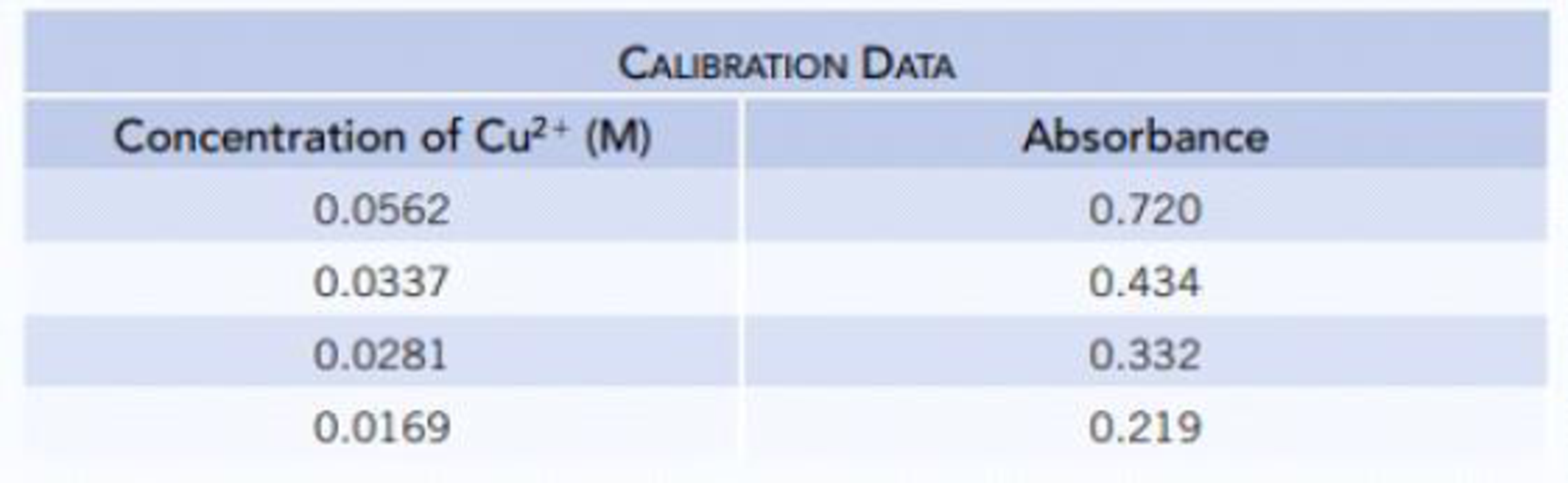# A solution of copper(II) ions has an absorbance of 0.418 when measured at 645 nm in a 1.0-cm cell. Using the following data, calculate the concentration of copper(II) ions in the unknown solution.### Chemistry & Chemical Reactivity

9th Edition
John C. Kotz + 3 others
Publisher: Cengage Learning
ISBN: 9781133949640

#### Solutions

Chapter
Section### Chemistry & Chemical Reactivity

9th Edition
John C. Kotz + 3 others
Publisher: Cengage Learning
ISBN: 9781133949640
Chapter 4.8, Problem 1CYU
Textbook Problem
63 views

## A solution of copper(II) ions has an absorbance of 0.418 when measured at 645 nm in a 1.0-cm cell. Using the following data, calculate the concentration of copper(II) ions in the unknown solution.Interpretation Introduction

Interpretation: The concentration of copper (II) ions in the given unknown solution should be calculated.

Concept Introduction:

• Concentration of solutions can be expressed in various terms; molarity is one such concentration expressing term.

### Explanation of Solution

First the given absorbance and the concentration data are plotted as follows,

When x=0y is 0.011, therefore the y intercept is 0.011.

From this the equation of straight line the concentration is calculated as follows,

y =  mx + cy =13x + 0

### Still sussing out bartleby?

Check out a sample textbook solution.

See a sample solution

#### The Solution to Your Study Problems

Bartleby provides explanations to thousands of textbook problems written by our experts, many with advanced degrees!

Get Started

Find more solutions based on key concepts
Name the following compounds. a Sn3(PO4)2 b NH4NO2 c Mg(OH)2 d NiSO3

General Chemistry - Standalone book (MindTap Course List)

The nervous system cannot use fat as fuel. T F

Nutrition: Concepts and Controversies - Standalone book (MindTap Course List)

Where in a eukaryotic cell is DNA found? How is that DNA organized?

Biology: The Dynamic Science (MindTap Course List)

Explain the different ways a giant molecular cloud can be triggered to contract.

Horizons: Exploring the Universe (MindTap Course List)

Review. A block of mass M = 0.450 kg is attached to one end of a cord of mass 0.003 20 kg: the other end of the...

Physics for Scientists and Engineers, Technology Update (No access codes included)

What benefits do estuaries provide? What are some threats to marine estuaries?

Oceanography: An Invitation To Marine Science, Loose-leaf Versin